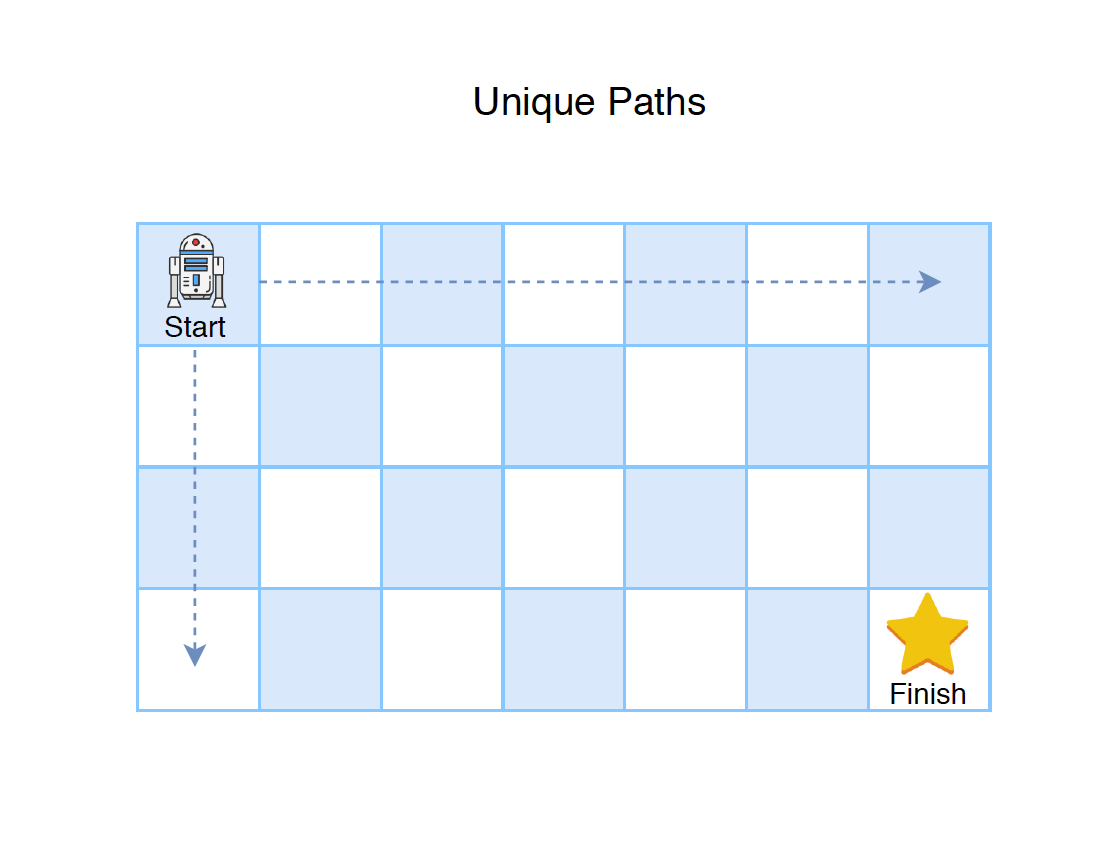# 1224. Algorithm - Matrix DPDP

Introduce dynamic programming.

Minimum Path Sum

• Follow up: print the path?

## 2. Matrix DP

### 2.1 Unique Paths

#### 2.1.1 Problem Description

A robot is located at the top-left corner of a m x n grid (marked ‘Start’ in the diagram below). The robot can only move either down or right at any point in time. The robot is trying to reach the bottom-right corner of the grid (marked ‘Finish’ in the diagram below). How many possible unique paths are there?#### 2.1.2 Solution with Matrix(Two-dimensional array)

// time: O(m*n), space: O(m*n)
public int uniquePathMatrix(int m, int n) {
if (m <= 0 || n <= 0) {
return 0;
}

int[][] dp = new int[m][n];
// Initialization
for (int i = 0; i < m; i++) {
dp[i] = 1;
}
for (int j = 0; j < n; j++) {
dp[j] = 1;
}
// Calculate dp[i][j]
for (int i = 1; i < m; i++) {
for (int j = 1; j < n; j++) {
dp[i][j] = dp[i][j - 1] + dp[i - 1][j];
}
}

return dp[m - 1][n - 1];
}

// time: O(m*n), space: O(m*n), without separated initialization
public int uniquePathMatrix2(int m, int n) {
if (m <= 0 || n <= 0) {
return 0;
}

int[][] dp = new int[m][n];
for (int i = 0; i < m; i++) {
for (int j = 0; j < n; j++) {
if (i == 0 || j == 0) {
dp[i][j] = 1;
} else {
dp[i][j] = dp[i][j - 1] + dp[i - 1][j];
}
}
}

return dp[m - 1][n - 1];
}

• Time complexity: $O(m*n)$
• Space complexity: $O(m*n)$

#### 2.1.3 Solution with One-dimensional Array

Use one-dimensional array instead of the matrix. Same time complexity, but space is reduced to $O(n)$.

// time: O(m*n), space: O(n)
public int uniquePathArray(int m, int n) {
if (m <= 0 || n <= 0) {
return 0;
}

int[] dp = new int[n];
for (int i = 0; i < m; i++) {
for (int j = 0; j < n; j++) {
if (j == 0) {
dp[j] = 1;
} else {
dp[j] = dp[j] + dp[j - 1];
}
}
}

return dp[n - 1];
}

// time: O(m*n), space: O(n), without checking the first column
public int uniquePath(int m, int n) {
if (m <= 0 || n <= 0) {
return 0;
}

int[] dp = new int[n];
dp = 1;
for (int i = 0; i < m; i++) {
for (int j = 0; j < n; j++) {
if (j > 0) {
dp[j] = dp[j] + dp[j - 1];
}
}
}

return dp[n - 1];
}

• Time complexity: $O(m*n)$
• Space complexity: $O(n)$

### 2.2 Define Matrix with Larger Size

When to define a dp matrix with m+1 and n+1? see question LeetCode 221 - Maximal Square.